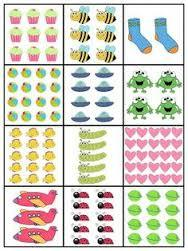3 years ago
brownt62
Save
Edit
Host a game
Live GameLive
Homework
Solo Practice
Practice10 QuestionsShow answers
• Question 1
120 secondsQ. Select the multiplication sentence for the following array:
3 x 3 = 9
5 x 9 = 45
2 x 3 = 6
1 x 6 = 6
• Question 2
120 secondsQ. Select the multiplication sentence for the follow example:
3 x 8 = 24
6 x 5 = 30
2 x 9 = 18
3 x 6 = 18
• Question 3
120 secondsQ. Which division sentence matches the array below?
8 ÷ 8 = 1
8 ÷ 4 = 2
6 ÷ 3 = 2
12 ÷ 4 = 3
• Question 4
120 secondsQ. Select the equation that would NOT be included in this fact family.
5 x 3 = 15
15 ÷ 5 = 3
5 x 3 = 15
3 x 6 = 18
• Question 5
120 secondsQ. Which division sentence describes this array?
4 x 2 = 8
8 ÷ 4 = 2
8 ÷ 8 = 1
8 ÷ 1 = 8
• Question 6
120 secondsQ. If I made these groups into an array they would have....
6 rows, 3 columns
2 rows, 6 columns
2 rows, three columns
You can't make it into an array.
• Question 7
120 secondsQ. Select the number sentence that best describes these unit blocks.
4 x 2 = 8
2 x 4 = 8
8 ÷ 4 = 2
4 ÷ 1 = 4
• Question 8
120 secondsQ. Each brown circle is a basket of apples. Choose the number sentence that best describes how many apples are in each basket.
4 x 4 = 16
4 x 1 = 4
4 x 0 = 0
0 x 0 = 0
• Question 9
120 secondsQ. Which number sentence best describes this group?
1 x 5 = 5
5 x 0 = 0
5 x 5 = 25
1 x 1 = 1
• Question 10
120 secondsQ. Does the array on the left mean the same thing as the groups on the right?